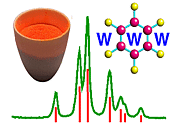Advanced Certificate in Powder Diffraction on the Web Chapter 6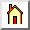Welcome Page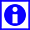Course Index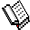Master Index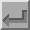Chapter Index

Chapter 6

Aims & Objectives

The aim of this chapter is to provide a basic theory, with or without mathematical proof, to diffraction in preparation for instruction on how to calculate the intensity of powder diffraction peaks. Specific objectives and points of counselling are:

• This chapter is the first of a two-part series: Diffraction Theory I (this chapter) and Diffraction Theory II (which will follow later). There are strong connections between these two: This week we go through the underlying theory of diffraction and then subsequently you will be shown how to apply it with specific examples.
• In Diffraction Theory I we start from a very basic point - the scattering of radiation, such as X-rays, from a single electron - and then build up gradually so that we eventually conclude with diffraction from a single crystal or crystalline powder. During the development of the theory there is unavoidably some considerable use made of mathematical tools such as exponentials, calculus, imaginary numbers and so on. However it is absolutely crucial here to emphasise that students who are not able to cope with this level of mathematics need not despair. The theory is developed in a way that emphasises the qualitative principles involved and then repeats the message in the appropriate mathematical language. A student who cannot follow the mathematical part can still remain in contact by making sure s/he at least understands the qualitative principles; obviously an understanding of the principles and the mathematics would be a bonus. Even for those who cannot follow the mathematics, you can still appreciate that the formulae often display a shape that reflects their qualitative meaning. In Diffraction II, the method will be explained in a prescriptive way that will enable all students to perform the necessary intensity calculations irrespective of mathematical grasp.
• To help the non-mathematical students, many diagrams have been used. Some of these diagrams are highly schematic and should not always be taken too literally, but rather as an aid to qualitative understanding.
• Apart from possibly one section on the Reciprocal Lattice (see below) Diffraction Theory I and II require that they be read sequentially and so links to all the various topics are not given here. The student is strongly advised to start at the beginning with scattering of X-rays by a single electron, and work linearly through the pages to the end.
• The idea of the reciprocal lattice falls naturally out from this theory. The reciprocal lattice is an important concept in crystallography though it is not vital in all aspects of powder diffraction. However, in recognition of its use in some of the later sections (e.g. unit-cell indexing) a quick essential guide to the reciprocal lattice (and the Ewald sphere) is given, together with pointers to its relevance for single crystals and crystalline powders. The use of reciprocal space in studies of amorphous/liquid materials is also briefly discussed.Welcome PageCourse IndexMaster IndexChapter Index